# NIO中的开发利器ByteBuffer源码解析

Posted by 皇甫嗷嗷叫 on 03-22，2021

## 主要API和属性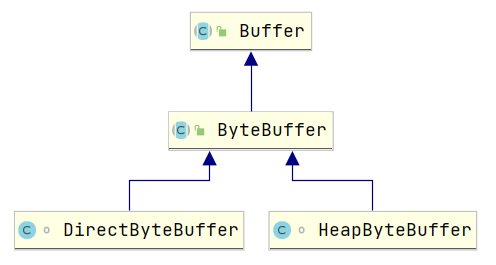### 主要属性

``````//指针标记
private int mark = -1;
//指针的当前位置
private int position = 0;
//翻转后界限
private int limit;
//最大容量
private int capacity;
//当为堆外内存的时候，内存的地址
``````

### 主要方法

``````//返回当前缓冲区的最大容量
public final int capacity() {return capacity;}
//返回当前的指针位置
public final int position() {return position;}
//返回当前的读写界限
public final int limit() {return limit;}
//标记当前指针位置
public final Buffer mark() {
mark = position;
return this;
}
//恢复当前指针位置
public final Buffer reset() {
int m = mark;
if (m < 0)
throw new InvalidMarkException();
position = m;
return this;
}
//清空缓冲区，注意这里并不会清空数据，只是将各项指标初始化，后续再写入数据就直接覆盖
public final Buffer clear() {
position = 0;
limit = capacity;
mark = -1;
return this;
}
//切换读写模式
public final Buffer flip() {
limit = position;
position = 0;
mark = -1;
return this;
}
//重新从头进行读写，初始化指针和标记位置
public final Buffer rewind() {
position = 0;
mark = -1;
return this;
}
//剩余可读可写的数量
public final int remaining() {return limit - position;}
//当前是否可读/可写
public final boolean hasRemaining() {return position < limit;}
//是不是只读的
//是不是支持数组访问
public abstract boolean hasArray();
//获取当前缓存的字节数组（当hasArray返回为true的时候）
public abstract Object array();
//是不是堆外缓冲区也就是直接缓冲区
public abstract boolean isDirect();
//取消缓冲区
final void discardMark() {mark = -1;}
``````

## 堆内缓冲

### 创建堆内缓冲区

java堆内缓冲区的默认实现是 HeapByteBuffer，但是这个对象是一个 default权限的类，你是无法直接创建的，只能通过JDK底层暴露的api来创建:

``````//1. 分配一个最大能够存储128个字节的堆内存
ByteBuffer heapRam = ByteBuffer.allocate(128);
//2. 或者直接初始化数据创建
ByteBuffer wrapBuffer = ByteBuffer.wrap("欢迎关注公众号:【源码学徒】 学习更多源码知识！".getBytes());
``````

### 堆内缓冲区API源码解析

#### 构造方法

``````public static ByteBuffer allocate(int capacity) {
if (capacity < 0) {
throw new IllegalArgumentException();
}
//创建一个堆内缓冲区
return new HeapByteBuffer(capacity, capacity);
}
``````

``````HeapByteBuffer(int cap, int lim) {
super(-1, 0, lim, cap, new byte[cap], 0);
}
``````

``````// mark = -1      pos = 0      lim = 128    cap = 128   hb = 字节数组对象    offset = 0
ByteBuffer(int mark, int pos, int lim, int cap, byte[] hb, int offset) {
super(mark, pos, lim, cap);
//前面创建的字节数组对象
this.hb = hb;
//保存一个偏移量 默认为0
this.offset = offset;
}
``````

``````Buffer(int mark, int pos, int lim, int cap) {
if (cap < 0){
throw new IllegalArgumentException("Negative capacity: " + cap);
}
//保存最大容量
this.capacity = cap;
//保存limit
limit(lim);
//保存pos指针位置
position(pos);
//........ 忽略无必要代码.........
}
``````#### put方法

``````heapRam.put("A".getBytes());
``````

``````public ByteBuffer put(byte[] src, int offset, int length) {
//先判断当前数据的长度是否超过可写长度了
// remaining() =  limit - position = 128 - 0
if (length > remaining()) {
throw new BufferOverflowException();
}
//hb还记得吗，就是我们再创建堆内缓冲区所创建的字节数组
//这里就是将我们的数据拷贝到从当前的指针位置开始的堆内缓存(hb字节数组)
System.arraycopy(src, offset, hb, ix(position()), length);
//将当前的指针位置 + 数据长度，我们本次写入的数据长度是1 那么当前的指针索引就是 1
position(position() + length);
return this;
}
``````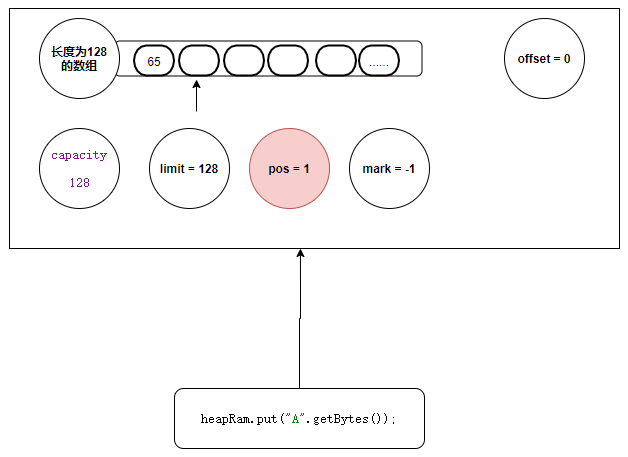``````heapRam.put("B".getBytes());
heapRam.put("C".getBytes());
heapRam.put("D".getBytes());
heapRam.put("E".getBytes());
``````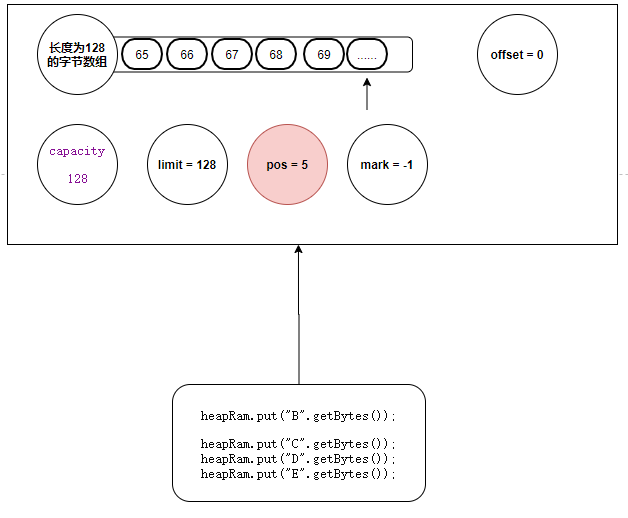#### get方法

``````byte b = heapRam.get();
System.out.println(new String(new byte[]{b}));
``````

``````heapRam.flip();
``````

``````public final Buffer flip() {
//将当前的指针位置赋值给limit
limit = position;
//读写指针复位
position = 0;
mark = -1;
return this;
}
``````

#### filp方法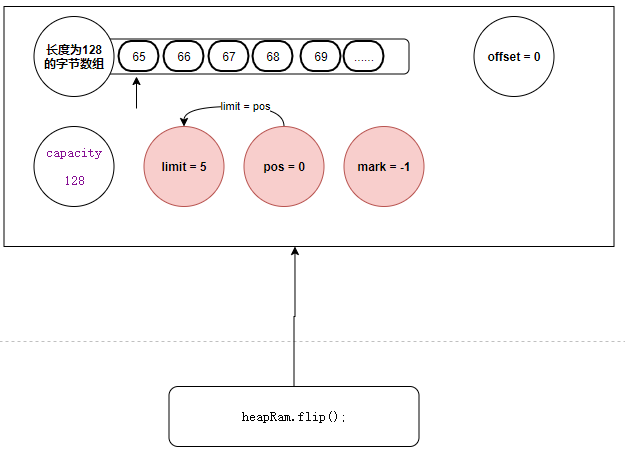``````byte b = heapRam.get();
System.out.println(new String(new byte[]{b}));
``````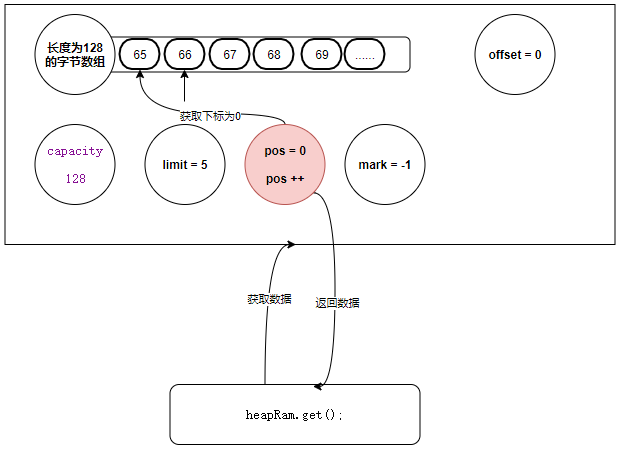``````public byte get() {
return hb[ix(nextGetIndex())];
}
``````

nextGetIndex：主要是判断当前指针是否超过了 limit的限制，同时自增指针位置

``````final int nextGetIndex() {
//limit的作用在这里被体现，判断你的读指针是不是读超了数据范围
if (position >= limit){
throw new BufferUnderflowException();
}
//返回读指针的位置，并自增1
return position++;
}
``````

get方法主要是直接返回字节数组某个下标的位置的字节数据！

``````byte[] bytes = new byte;
heapRam.get(bytes);
System.out.println(new String(bytes));
``````

``````public ByteBuffer get(byte[] dst) {
return get(dst, 0, dst.length);
}
``````
``````public ByteBuffer get(byte[] dst, int offset, int length) {
checkBounds(offset, length, dst.length);
if (length > remaining())
throw new BufferUnderflowException();
//将数据拷贝至我们传递的字节数组中
System.arraycopy(hb, ix(position()), dst, offset, length);
//读指针位置+我们要读取的长度
position(position() + length);
return this;
}
``````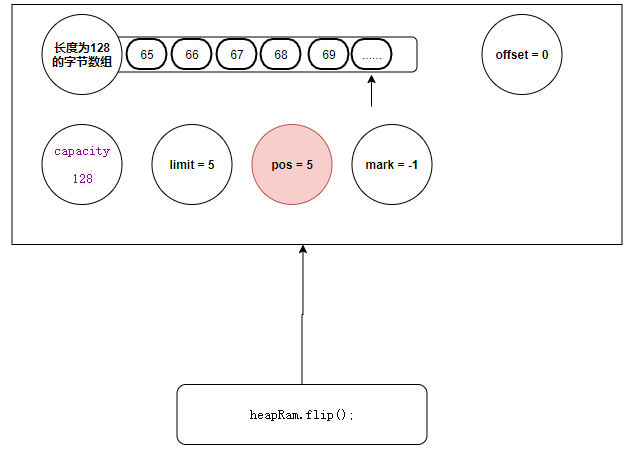``````if (length > remaining())
throw new BufferOverflowException();
``````

``````public final int remaining() {
return limit - position;
}
``````

#### clear方法

``````heapRam.clear();
``````
`````` public final Buffer clear() {
//读写指针归零
position = 0;
//limit初始化为初始状态
limit = capacity;
//标记初始化为初始状态
mark = -1;
return this;
}
``````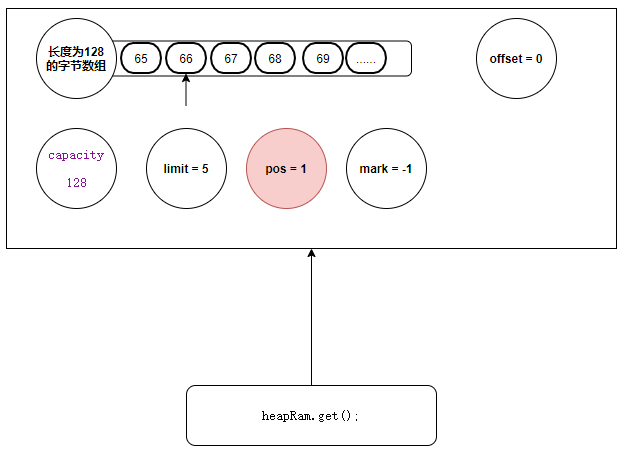#### mark方法

``````heapRam.mark();
``````
``````public final Buffer mark() {
//记录当前读指针的位置
mark = position;
return this;
}
``````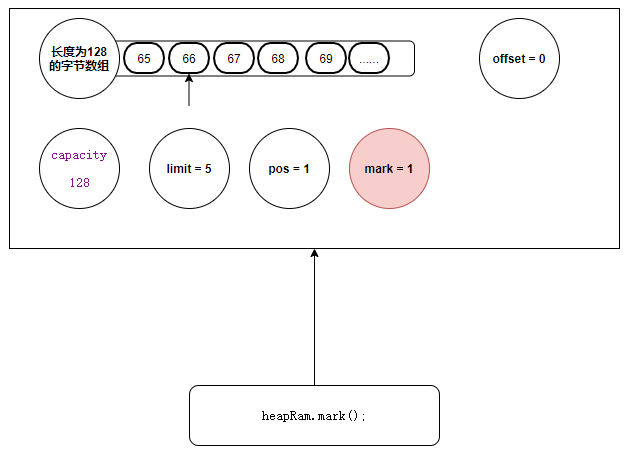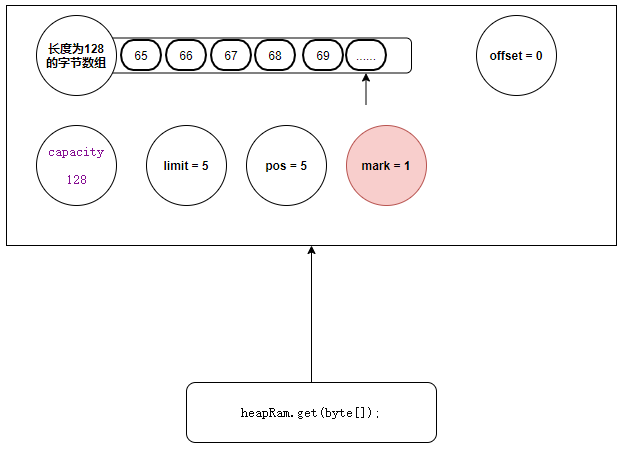#### reset方法

``````heapRam.reset();
``````
``````public final Buffer reset() {
//获取当前标记的位置
int m = mark;
if (m < 0)
//如果标记位为负数，就证明没有进行标记过直接报错
throw new InvalidMarkException();
//然后将标记位置赋值给当前的指针位置
position = m;
return this;
}
``````#### rewind方法

``````heapRam.rewind();
``````
``````public final Buffer rewind() {
//回复读写指针为0
position = 0;
//废弃标记位置
mark = -1;
return this;
}
``````

rewind方法是直接返回的 缓冲区的头部，同时废弃标记的位置 ！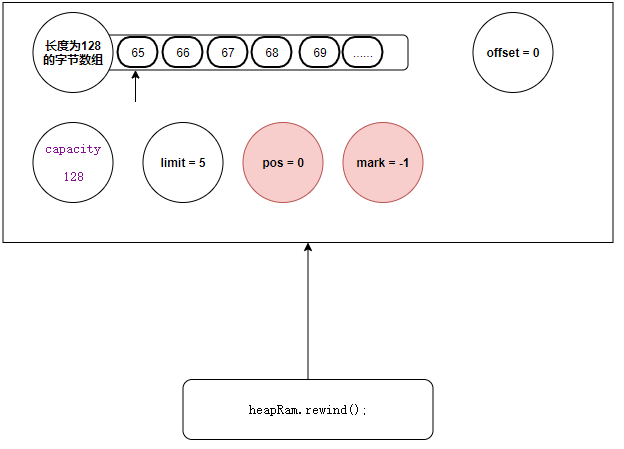## 堆外缓冲区

### 创建堆外缓冲区

``````//1. 分配一个最大能够存储128个字节的堆外内存
ByteBuffer byteBuffer = ByteBuffer.allocateDirect(128);
``````

### jvm如何操作堆外内存

``````public static void main(String[] args) throws NoSuchFieldException, IllegalAccessException {
//获取JDK底层的操作物理内存的工具类
Field theUnsafe = Unsafe.class.getDeclaredField("theUnsafe");
theUnsafe.setAccessible(true);
Unsafe o = (Unsafe) theUnsafe.get(null);
//从物理内存分配一块128的内存
//获取字节数组的一个基本偏移  数组基本偏移
long arrayBaseOffset = (long)o.arrayBaseOffset(byte[].class);
byte[] bytes = "欢迎关注公众号:【源码学徒】 学习更多源码知识！".getBytes();

//向物理内存复制一段数据
// 数据源     数据的基本偏移    目标数据源     要复制到的内存地址    复制数据的长度
//从物理机将数据拷贝回JVM内存中
byte[] copy = new byte[bytes.length];
// 数据源     物理地址    目标数据源     数组基本偏移量    复制数据的长度
//释放内存
System.out.println(new String(copy));
}
``````

### 堆外缓冲区Api源码解析

#### 构造方法

``````public static ByteBuffer allocateDirect(int capacity) {
return new DirectByteBuffer(capacity);
}
``````

``````DirectByteBuffer(int cap) {

super(-1, 0, cap, cap);
//......忽略其他代码..........

long base = 0;
try {
//从物理内存分配一块指定大小的内存 并返回当前分配内存的地址
base = unsafe.allocateMemory(size);
} catch (OutOfMemoryError x) {
Bits.unreserveMemory(size, cap);
throw x;
}
//初始化内存
unsafe.setMemory(base, size, (byte) 0);
//判断是否对其的页面
if (pa && (base % ps != 0)) {
// 向上对其页面 并保存地址
address = base + ps - (base & (ps - 1));
} else {
//保存地址
}
//这个极其重要，是JVM管理堆外内存的重要方法
cleaner = Cleaner.create(this, new Deallocator(base, size, cap));
att = null;
}
``````

``````//      -1   0   128    128
MappedByteBuffer(int mark, int pos, int lim, int cap) {
//继续调用父类
super(mark, pos, lim, cap);
this.fd = null;
}
``````
``````// -1   0   128   128
ByteBuffer(int mark, int pos, int lim, int cap) {
//在往上
this(mark, pos, lim, cap, null, 0);
}
``````
``````//   -1   0   128   128   null    0
ByteBuffer(int mark, int pos, int lim, int cap, byte[] hb, int offset) {
super(mark, pos, lim, cap);
this.hb = hb;
this.offset = offset;
}
``````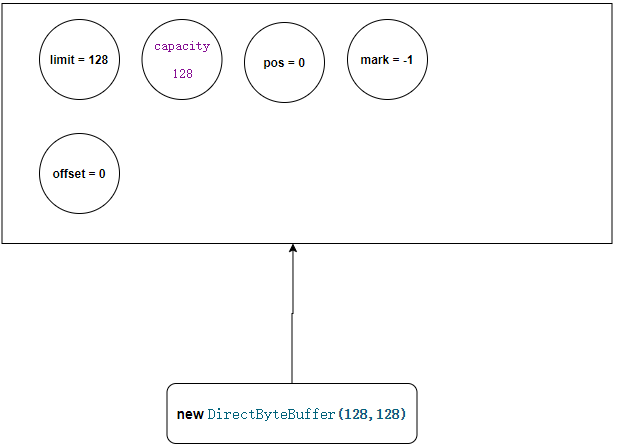``````base = unsafe.allocateMemory(size);
``````

``````unsafe.setMemory(base, size, (byte) 0);
``````

``````address = base;
``````

``````cleaner = Cleaner.create(this, new Deallocator(base, size, cap));
``````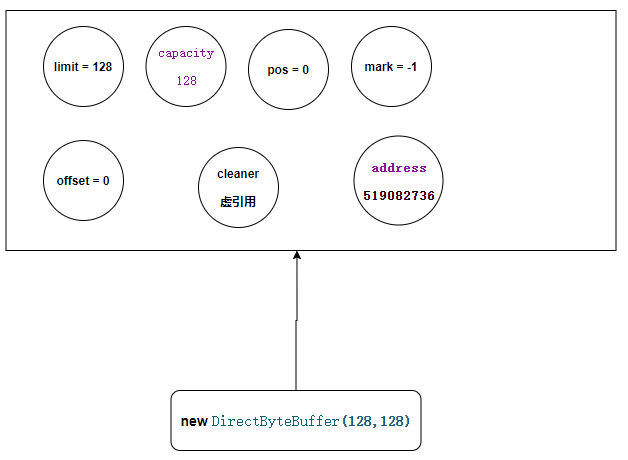#### put方法

``````byteBuffer.put("ABCDE".getBytes());
``````

``````public final ByteBuffer put(byte[] src) {
return put(src, 0, src.length);
}
``````
``````public ByteBuffer put(byte[] src, int offset, int length) {

if (((long)length << 0) > Bits.JNI_COPY_FROM_ARRAY_THRESHOLD) {
//检查越界
checkBounds(offset, length, src.length);
//获取当前的读写指针
int pos = position();
//获取当前的学些界限
int lim = limit();
//判断是否超过读写界限
assert (pos <= lim);
//计算剩余空间
int rem = (pos <= lim ? lim - pos : 0);
//判断写入数据是否大于剩余空间
if (length > rem) {
throw new BufferOverflowException();
}
//向物理内存拷贝数据
Bits.copyFromArray(src, arrayBaseOffset, (long)offset << 0, ix(pos), (long)length << 0);
//重新计算当前的读写指针
position(pos + length);
} else {
super.put(src, offset, length);
}
return this;

}
``````